## 1. Introduction

Devices on a network are identified by a unique IP address, consisting of two parts: the network ID and the host ID.

However, determining the network ID and host ID from an IP address can be a little tricky, especially if you don’t know the subnet mask of the network. The subnet mask determines which bits in the IP address are used to identify the network and host.

In this tutorial, we’ll show you how to figure out the subnet ID and host ID from an IP address.

IP addressing is a method of assigning unique addresses to devices connected to the internet. It was the first system developed for IP addressing and is still used in networks today. The representation of a 32-bit IP address as a sequence of 32 binary digits or bits is usually written in decimal notation, with each of the four octets separated by a period (.) symbol.

The following figure shows an example of an IP address conversion: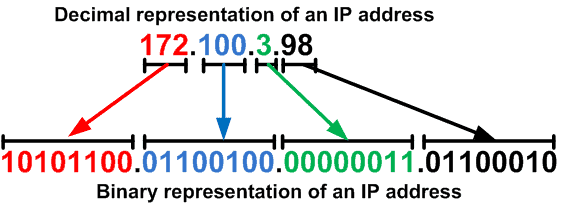An example of an IP address conversion

In decimal notation, each octet represents a number between 0 and 255. The first octet is the most significant and the fourth octet is the least significant. As clearly seen from the figure above, converting each octet from decimal to binary obtains the binary representation of the IP address, or vice versa.

The standard organization divides IP addresses into five classes: A, B, C, D, and E. Each class has a predefined range of IP addresses that can be assigned to devices.

A Class A address is a type of IP address used for large networks. Hence, the address’s first octet, which ranges from 1 to 126 in decimal notation, identifies the Class A address. Therefore, the first bit of the first octet is always 0, indicating that this is a Class A address.

In binary notation, a Class A address has the format: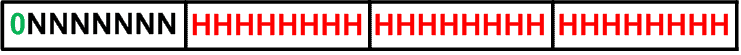The NNNNNNN represents the network ID, while the remaining HHHHHHHH.HHHHHHHH.HHHHHHHH bits represent the host ID.

Since the network ID takes up 7 bits, the maximum number of Class A networks that is 2^7 – 2, or 126. The maximum number of hosts per network is 2^24 – 2, or approximately 16.7 million. Thus, large organisations, such as multinational corporations or government entities, typically use Class A addresses that require a large number of hosts on a single network.

A Class B address is a type of IP address used for medium-sized networks. Hence, the address’s first two octets, which range from 128 to 191 in decimal notation, identify the Class B address. Therefore, the first two bits of the first octet are always 10, indicating that this is a Class B address.

In binary notation, a Class B address has the format: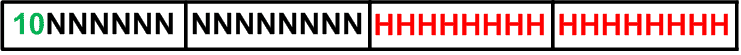The NNNNNNN.NNNNNNNN represents the network ID, while the remaining HHHHHHHH.HHHHHHHH bits represent the host ID.

Since the network ID takes up 14 bits, the maximum number of Class B networks that is 2^14, or approximately 16,000. The maximum number of hosts per network is 2^16 – 2, or 65,534. Therefore, moderate organizations that require a moderate number of hosts on a single network, such as universities or large companies with multiple locations, typically use Class B addresses.

A Class C address is a type of IP address used for small-sized networks. Hence, the address’s first three octets, which range from 192 to 223 in decimal notation, identify the Class C address. Therefore, the first three bits of the first octet are always 110, indicating that this is a Class C address.

In binary notation, a Class C address has the format: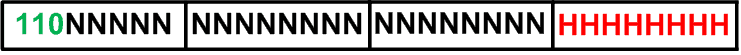The NNNNNN.NNNNNNNN.NNNNNNNN represents the network ID, while the remaining HHHHHHHH bits represent the host ID.

Since the network ID takes up 21 bits, the maximum number of Class C networks is 2^21, or approximately 2 million. On the other hand, the maximum number of hosts per network is 2^8 – 2, or 254. Small organisations or network subnets within larger organizations typically use Class C addresses. In addition, people commonly use Class C addresses for home networks and small office networks.

### 2.4. Class D and E Addresses

Class D and Class E addresses are two other types of IP addresses that are not commonly used for network addressing purposes.

In computer networking, multicast addressing is a technique used to send data packets to a group of hosts on a network. Hence, these hosts are identified by a multicast group address, which is represented by a Class D IP address. A Class D address has the first four bits of the first octet set to 1110, while the remaining bits are used to identify the multicast group.

In binary notation, a Class D address has the format: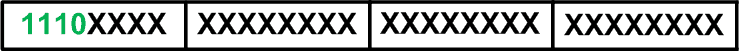The X bits represent the multicast group ID. On the other hand, the first octet sets the first four bits to 1111, which identifies a Class E address. Moreover, future use or experimental purposes reserve the Class E addresses.

In binary notation, a Class E address has the format: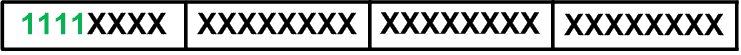Before we dive into figuring out the subnet ID and host ID, let’s first understand subnet masks. A subnet mask is a 32-bit number that is used to divide an IP address into two parts: the network ID and the host ID.

The subnet mask consists of a series of 1s and 0s. The 1s indicate which bits in the IP address belong to the network ID, while the 0s indicate which bits belong to the host ID. For example, a subnet mask of 255.255.255.0 means that the first three IP address octets belong to the network ID, while the last octet belongs to the host ID. The following table summarises the subnet mask for each class.The CIDR notation is a shorthand way of representing the subnet mask, where the number after the slash (/) represents the number of bits used for the network ID.

The number of available IP addresses per network decreases as the subnet mask gets larger (i.e., fewer bits for the host ID). However, the number of available networks increases. Conversely, the number of available IP addresses per network increases as the subnet mask gets smaller (i.e., more bits for the host ID). But the number of available networks decreases.

It’s important to choose the right subnet mask for a network to ensure that there are enough IP addresses for the devices on the network. One must choose the appropriate subnet mask for a network to ensure that there are enough IP addresses for the devices on the network without wasting any IP addresses.

### 3.1. Determining the Subnet ID and Host ID

Now that we understand subnet masks let’s figure out the subnet ID and host ID from an IP address using the subnet mask. Suppose we have a Class C IP address 192.168.1.50 with a subnet mask of 255.255.255.0.

First, we need to convert the IP address and subnet mask to binary. The following figure shows a binary representation of 192.168.1.50 with a subnet mask of 255.255.255.0: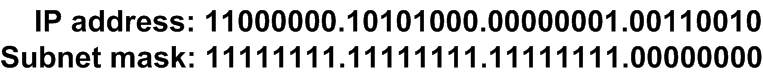Next, we perform a bitwise AND operation between the IP address and the subnet mask: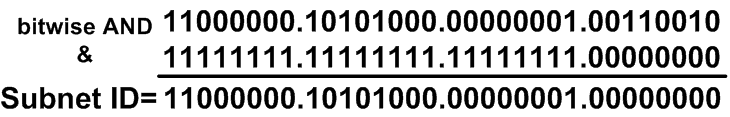Therefore, the resulting binary number is the subnet ID (11000000.10101000.00000001.00000000), which we need to convert back to decimal form. Thus 192.168.1.0 is the subnet ID and the remaining bits in the IP address (00110010) are the host ID, which we also need to convert back to decimal form: Host ID: 50. Therefore, the IP address 192.168.1.50 belongs to the network 192.168.1.0 with a host ID of 50.

Let’s have a look at more examples of this.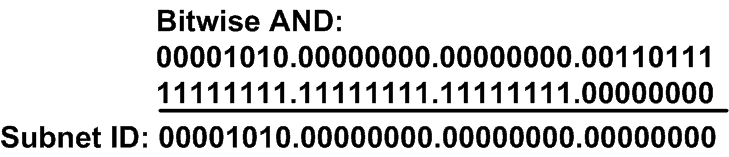Therefore: Subnet ID: 10.0.0.0 and Host ID:55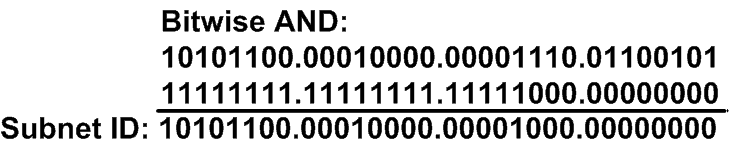Therefore: Subnet ID: 172.16.8.0 and Host ID:14.101

Similarly to the previous example, we perform a bitwise AND operation between the IP address and the subnet mask to determine the subnet ID and host ID, for example, 1 and 2. This calculation shows that the subnet ID, for example 1, is 10.0.0.0 and the host ID is 55. On the other hand, the subnet and host IDs are 172.16.8.0 and 14.101, for example 2, respectively.

## 4. Conclusion

Understanding how to figure out the subnet ID and host ID from an IP address is important for anyone working with computer networks. By using the subnet mask, you can easily divide an IP address into two parts and identify the network and host to which a device belongs.

While the process may seem a little complex at first, with a bit of practice, you’ll be able to determine the subnet ID and host ID in no time.